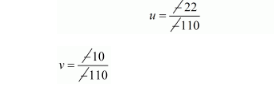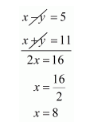# The boat goes 30 km upstream and 44 km downstream in 10 hours.

Question:

The boat goes 30 km upstream and 44 km downstream in 10 hours. In 13 hours, it can go 40 km upstream and 55 km downstream. Determine the speed of stream and that of the boat in still water.

Solution:

Let the speed of the boat in still water be x km/hr and the speed of the stream be y km/hr

Speed upstream $=(x-y) \mathrm{km} / \mathrm{hr}$

Speed down stream $=(x+y) \mathrm{km} / \mathrm{hr}$

Now,

Time taken to cover $30 \mathrm{~km}$ upstream $=\frac{30}{x-y}$ hrs

Time taken to cover $44 \mathrm{~km}$ down stream $=\frac{44}{x+y}$ hrs

But total time of journey is 10 hours

$\frac{30}{x-y}+\frac{44}{x+y}=10 \cdots(i)$

Time taken to cover $40 \mathrm{~km}$ upstream $=\frac{40}{x-y} h r s$

Time taken to cover $55 \mathrm{~km}$ down stream $=\frac{55}{x+y} h r s$

In this case total time of journey is given to be 13 hours

Therefore, $\frac{40}{x-y}+\frac{55}{x+y}=13$$\ldots$ (ii)

Putting $\frac{1}{x-y}=u$ and $\frac{1}{x+y}=v$ in equation $(i)$ and $(i i)$ we get

$30 u+44 v=10$

$40 u+55 v=10$

$30 u+44 v-10=0 \cdots(i i i)$

$40 u+55 v-13=0 \cdots(i v)$

Solving these equations by cross multiplication we get

$\frac{u}{44 \times-13-55 \times-10}=\frac{-v}{30 \times-13-40 \times-10}=\frac{1}{30 \times 55-40 \times 44}$

$\frac{u}{-572+550}=\frac{-v}{-390+400}=\frac{1}{1650-1760}$

$\frac{u}{-22}=\frac{-v}{10}=\frac{1}{-110}$$u=\frac{2}{10}$ and $v=\frac{1}{11}$

Now,

$u=\frac{2}{10}$

$\frac{1}{x-y}=\frac{2}{10}$

$1 \times 10=2(x-y)$

$10=2 x-2 y \div 2$

$5=x-y \cdots(v)$

$v=\frac{1}{11}$

$\frac{1}{x+y}=\frac{1}{11}$

$1 \times 11=1(x+y)$

$11=x+y \cdots(v i)$

By solving equation $(v)$ and $(v i)$ we get,Substituting $x=8$ in equation $(v i)$ we get,

$x+y=11$

$8+y=11$

$y=11-8$

$y=3$

Hence, speed of the boat in still water is $8 \mathrm{~km} / \mathrm{hr}$

Speed of the stream is $3 \mathrm{~km} / \mathrm{hr}$